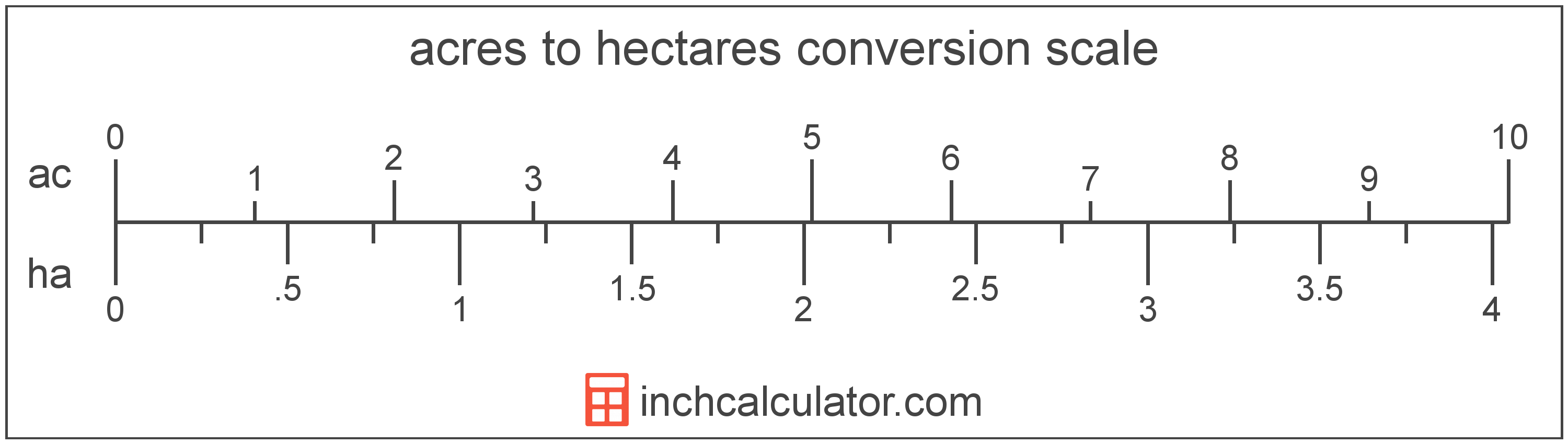Convert Hectares to Acres

Enter the area in hectares below to get the value converted to acres.

Results in Acres:1 ha = 2.471054 ac
Do you want to convert acres to hectares?

How to Convert Hectares to AcresTo convert a hectare measurement to a acre measurement, multiply the area by the conversion ratio. One hectare is equal to 2.471054 acres, so use this simple formula to convert:

acres = hectares × 2.471054

The area in acres is equal to the hectares multiplied by 2.471054.

For example, here's how to convert 5 hectares to acres using the formula above.
5 ha = (5 × 2.471054) = 12.355269 ac

Hectares and acres are both units used to measure area. Keep reading to learn more about each unit of measure.

Hectares

One hectare is equal to 10,000 square meters, or the area of a square with 100 meter sides.

The hectare is an SI accepted unit for area for use with the metric system. In the metric system, "hecto" is the prefix for 102. Hectares can be abbreviated as ha, for example 1 hectare can be written as 1 ha.

Acres

One acre is defined as the area equal to a space that is one chain (66 ft) by one furlong (660 ft), or 10 square chains. That's equal to 43,560 square feet for those unfamiliar with those units of measure.

The acre is a US customary and imperial unit of area. Acres can be abbreviated as ac, for example 1 acre can be written as 1 ac.

Try our acreage calculator to find the area of a plot of land in acres by locating the boundaries on a map.

Hectare Measurements and Equivalent Acre Conversions

Common hectare values converted to the equivalent acre value
Hectares Acres
1 ha 2.4711 ac
2 ha 4.9421 ac
3 ha 7.4132 ac
4 ha 9.8842 ac
5 ha 12.36 ac
6 ha 14.83 ac
7 ha 17.3 ac
8 ha 19.77 ac
9 ha 22.24 ac
10 ha 24.71 ac
11 ha 27.18 ac
12 ha 29.65 ac
13 ha 32.12 ac
14 ha 34.59 ac
15 ha 37.07 ac
16 ha 39.54 ac
17 ha 42.01 ac
18 ha 44.48 ac
19 ha 46.95 ac
20 ha 49.42 ac
21 ha 51.89 ac
22 ha 54.36 ac
23 ha 56.83 ac
24 ha 59.31 ac
25 ha 61.78 ac
26 ha 64.25 ac
27 ha 66.72 ac
28 ha 69.19 ac
29 ha 71.66 ac
30 ha 74.13 ac
31 ha 76.6 ac
32 ha 79.07 ac
33 ha 81.54 ac
34 ha 84.02 ac
35 ha 86.49 ac
36 ha 88.96 ac
37 ha 91.43 ac
38 ha 93.9 ac
39 ha 96.37 ac
40 ha 98.84 ac

More Hectare Area Conversions

US Customary
Convert to Square Miles
1 ha is equal to 0.003861 square miles
Convert to Square Yards
1 ha is equal to 11,959.9 square yards
Convert to Square Feet
1 ha is equal to 107,639.1 square feet
Convert to Square Inches
1 ha is equal to 15,500,031 square inches
SI Units
Convert to Square Kilometers
1 ha is equal to 0.01 square kilometers
Convert to Square Meters
1 ha is equal to 10,000 square meters
Convert to Square Centimeters
1 ha is equal to 100,000,000 square centimeters
Convert to Square Millimeters
1 ha is equal to 10,000,000,000 square millimeters
Other Metric Units
Convert to Ares
1 ha is equal to 100 ares